A combined-cycle power plant comprises gas turbines, steam turbines, and heat recovery steam generators.

In this type of plant, the electricity is generated by gas and steam turbines combined in one cycle. Then, it is transferred from one turbine to another.

This process entails the emission of some gas pollutants such as NOx (nitrogen oxides), harmful to our health. Predicting peaks in such emissions might give us a chance to put preventive actions in motion.

This example models the NOx levels to give us essential information about the power plant’s emissions and reduce them.

### Contents

This example is solved with Neural Designer. You can use the free trial to understand how the solution is achieved step by step.

## 1. Application type

This is an approximation project since the variable to be predicted is continuous (NOx emission levels).

The fundamental goal here is to model pollutant emissions as a function of the environmental and control variables.

## 2. Data set

The data set contains three concepts:

• Data source.
• Variables.
• Instances.

The data file power_plant_gas_emissions.csv contains 36733 samples with 11 variables aggregated over one hour from a gas turbine located in Turkey’s northwestern region between the years 2011-2015.

The variables, or features, are the following:

• ambient_temperature, in degrees Celsius.
• ambient_pressure, in millibars.
• ambient_humidity, as a percentage.
• air_filter_difference_pressure, difference of pressure in the air filter, in millibars.
• gas_turbine_exhaust_pressure, pressure of the combustion chamber exhaust gases, in millibar.
• turbine_inlet_temperature, temperature of the combustion chamber exhaust gases as they enter the turbine unit, in degrees Celsius.
• turbine_after_temperature, temperature of the combustion chamber exhaust gases as they exit the turbine unit, in degrees Celsius.
• compressor_discharge_pressure, pressure of the gases expelled by the compressor, in millibars.
• turbine_energy_yield, total energy yielded by the turbine in an hour, in Megawatts per hour.
• NOx, concentration of nitrogen oxides, in milligrams per cubic meter.

Our target variable will be the last one, NOx.

The instances are divided into training, selection, and testing subsets. They represent 60%, 20%, and 20% of the original instances, respectively, and are split at random.

Calculating the data distributions helps us check for the correctness of the available information and detect anomalies. The following chart shows the histogram for the NOx variable: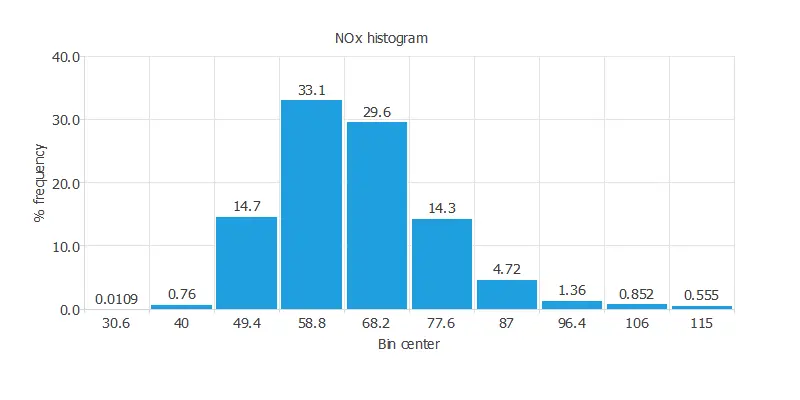We can see a normal distribution in the NOx histogram.

It is also interesting to look for dependencies between a single input and single target variables. To do that, we can plot an inputs-targets correlations chart.

For the NOx we have: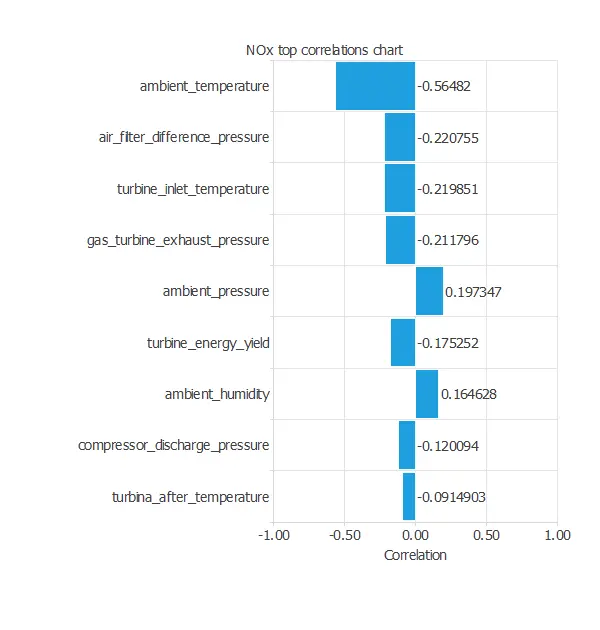In this case, the highest correlation is with the ambient temperature (the higher the temperature is, the less NOx is emitted).

Next, we plot a scatter chart for the most significant correlations for our target variable.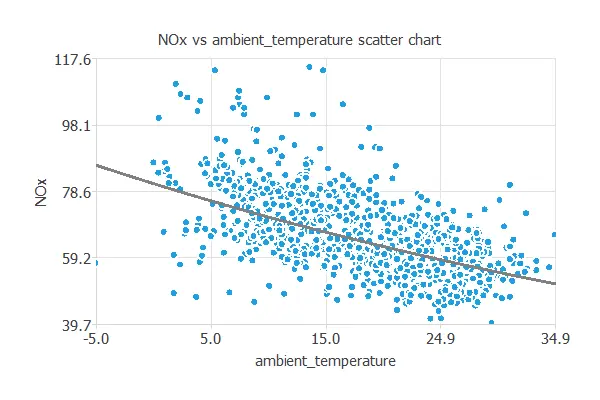As we saw earlier, the higher the ambient temperature, the less gas is emitted by the power plant.

## 3. Neural network

The second step is to build a neural network that represents the approximation function. Approximation problems, it is usually composed by:

• Scaling layer.
• Perceptron layers.
• Unscaling layer.

The neural network has nine inputs (ambient temperature, ambient pressure, ambient humidity, air filter difference pressure, gas turbine exhaust pressure, turbine inlet temperature, turbine after temperature, compressor discharge pressure, and turbine energy yield) and one output (NOx).

The scaling layer contains the statistics of the inputs. We use the automatic setting for this layer to accommodate the best scaling technique for our data.

We use 2 perceptron layers here:

The unscaling layer contains the statistics of the outputs. We use the automatic method as before.

The next graph represents the neural network for this example.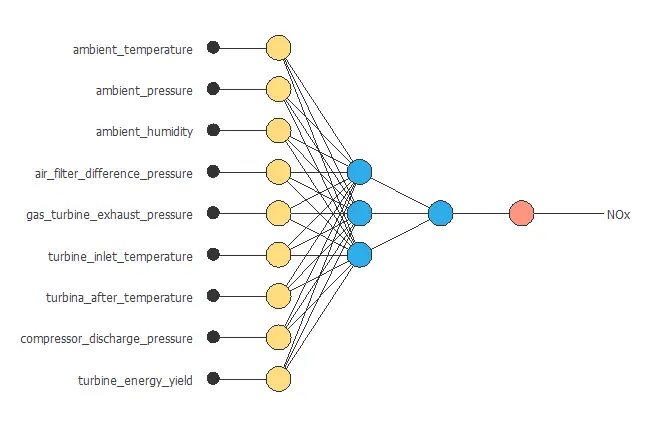## 4. Training strategy

The fourth step is to select an appropriate training strategy. It is composed of two parameters:

• Loss index.
• Optimization algorithm.

The loss index defines what the neural network will learn. It is composed of an error term and a regularization term.

The error term chosen is the normalized squared error. It divides the squared error between the outputs from the neural network and the targets in the data set by its normalization coefficient. If the normalized squared error has a value of 1, then the neural network is predicting the data ‘in the mean’, while a value of zero means a perfect prediction of the data. This error term does not have any parameters to set.

The regularization term is the L2 regularization. It is applied to control the complexity of the neural network by reducing the value of the parameters. We use a weak weight for this regularization term.

The optimization algorithm is in charge of searching for the neural network parameters that minimize the loss index. Here we chose the quasi-Newton method as optimization algorithm.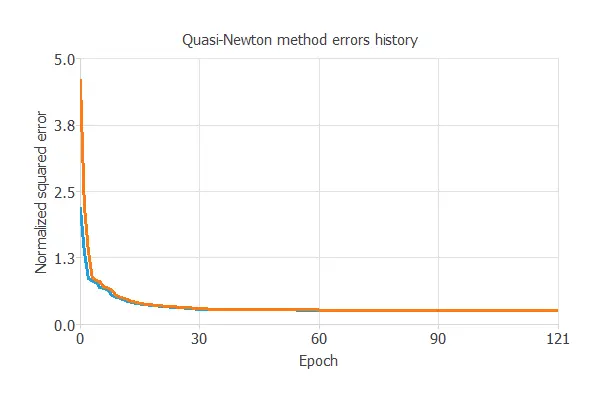The following chart shows how the training (blue) and selection (orange) errors decrease with the epochs during the training process. The final values are training error = 0.260 NSE and selection error = 0.263 NSE, respectively.

## 5. Model selection

The objective of model selection is to find the network architecture with the best generalization properties. We want to improve the final selection error obtained before (0.263 NSE).

The best selection error is achieved using a model with the most appropriate complexity to produce a good data fit. Order selection algorithms are responsible for finding the optimal number of perceptrons in the neural network.

The following chart shows the results of the incremental order algorithm. The blue line plots the final training error as a function of the number of neurons. The orange line plots the final selection error as a function of the number of neurons.As we can see, the final training error continuously decreases with the number of neurons. However, the final selection error takes a minimum value at some point. Here, the optimal number of neurons is 8, corresponding to a selection error of 0.224 NSE.

The following figure shows the optimal network architecture for this application.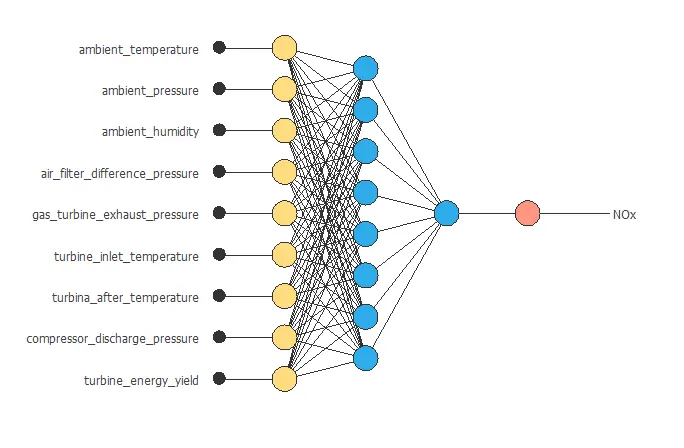## 6. Testing analysis

The purpose of the testing analysis is to validate the generalization capabilities of the neural network. We use the testing instances in the data set, which have never been used before.

A standard testing method in approximation applications is to perform a linear regression analysis between the predicted and the real pollutant level values.For a perfect fit, the correlation coefficient R2 would be 1. As we have R2 = 0.889, the neural network predicts the testing data quite well.

## 7. Model deployment

In the model deployment phase, the neural network is used to predict outputs for inputs that it has never seen.

We can calculate the neural network outputs for a given set of inputs:

• ambient_temperature: 17.713 degrees Celsius.
• ambient_pressure: 1013.07 millibars.
• ambient_humidity: 77.867 %.
• air_filter_difference_pressure: 3.926 millibars.
• gas_turbine_exhaust_pressure: 25.56 millibars.
• turbine_inlet_temperature: 1081.44 degrees Celsius.
• turbine_after_temperature: 546.161 degrees Celsius.
• compressor_discharge_pressure: 133.506 millibars.
• turbine_energy_yield: 12.061 Megawatts per hour.
• NOx: 67.518 milligrams per cubic meter.

We can also use Response Optimization. The objective of the response optimization algorithm is to exploit the mathematical model to look for optimal operating conditions. Indeed, the predictive model allows us to simulate different operating scenarios and adjust the control variables to improve efficiency.

An example is to minimize gas turbine exhaust pressure while maintaining the NOx level below the desired value.

The next table resumes the conditions for this problem.

Variable name Condition
Ambient temperature None
Ambient pressure None
Ambient humidity None
Air filter difference pressure None
Gas turbine exhaust pressure Minimize
Turbine inlet temperature None
Turbine after temperature None
Compressor discharge pressure None
Turbine energy yield None
NOx Less than or equal to 80

The next list shows the optimum values for previous conditions.

• ambient_temperature: 21.2745 degrees Celsius.
• ambient_pressure: 1033.75 millibars.
• ambient_humidity: 53.3598 %.
• air_filter_difference_pressure: 6.02753 millibars.
• gas_turbine_exhaust_pressure: 17.8926 millibars.
• turbine_inlet_temperature: 1008.55 degrees Celsius.
• turbine_after_temperature: 515.503 degrees Celsius.
• compressor_discharge_pressure: 136.975 millibars.
• turbine_energy_yield: 15.0443 Megawatts per hour.
• NOx: 40.7311 milligrams per cubic meter.

Directional outputs plot the neural network outputs through some reference points.

The next list shows the reference points for the plots.

• ambient_temperature: 17.713 degrees Celsius.
• ambient_pressure: 1013.07 millibars.
• ambient_humidity: 77.867 %.
• air_filter_difference_pressure: 3.926 millibars.
• gas_turbine_exhaust_pressure: 25.56 millibars.
• turbine_inlet_temperature: 1081.44 degrees Celsius.
• turbine_after_temperature: 546.161 degrees Celsius.
• compressor_discharge_pressure: 133.506 millibars.
• turbine_energy_yield: 12.061 Megawatts per hour.

We can see here how the turbine inlet temperature affects NOx emissions: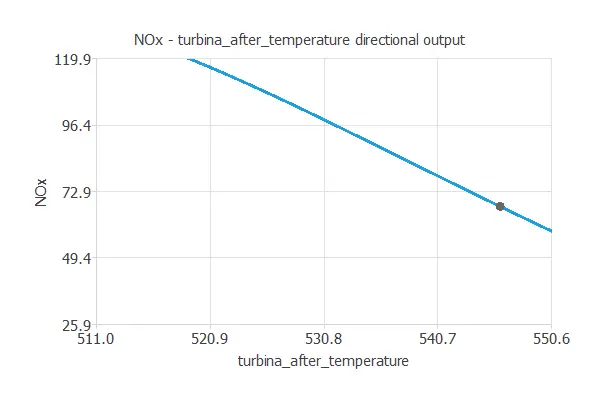Decreasing the turbine inlet temperature decreases NOx emissions.

This insight into our model can help us take preventive action against pollutant emissions. For example, if we calculate the neural network outputs again, simply decreasing the turbine inlet temperature by 10 degrees Celsius leads to a decrease in NOx levels from the previous 64.759 to 54.388 milligrams per cubic meter, which corresponds to a 19.45% decrease of this pollutant.

We are using the turbine inlet temperature to reduce NOx levels because it might be easy to change its value, but, using directional outputs, we could analyze any of our variables for this purpose.

The mathematical expression represented by the predictive model is displayed next:

scaled_ambient_temperature = ambient_temperature*(1+1)/(37.10300064-(-6.234799862))+6.234799862*(1+1)/(37.10300064+6.234799862)-1;
scaled_ambient_pressure = ambient_pressure*(1+1)/(1036.599976-(985.8499756))-985.8499756*(1+1)/(1036.599976-985.8499756)-1;
scaled_ambient_humidity = ambient_humidity*(1+1)/(100.1999969-(24.08499908))-24.08499908*(1+1)/(100.1999969-24.08499908)-1;
scaled_air_filter_difference_pressure = (air_filter_difference_pressure-(3.925529957))/0.7738839984;
scaled_gas_turbine_exhaust_pressure = gas_turbine_exhaust_pressure*(1+1)/(40.7159996-(17.69799995))-17.69799995*(1+1)/(40.7159996-17.69799995)-1;
scaled_turbine_inlet_temperature = turbine_inlet_temperature*(1+1)/(1100.900024-(1000.799988))-1000.799988*(1+1)/(1100.900024-1000.799988)-1;
scaled_turbina_after_temperature = turbina_after_temperature*(1+1)/(550.6099854-(511.0400085))-511.0400085*(1+1)/(550.6099854-511.0400085)-1;
scaled_compressor_discharge_pressure = compressor_discharge_pressure*(1+1)/(179.5-(100.0199966))-100.0199966*(1+1)/(179.5-100.0199966)-1;
scaled_turbine_energy_yield = turbine_energy_yield*(1+1)/(15.1590004-(9.851799965))-9.851799965*(1+1)/(15.1590004-9.851799965)-1;

perceptron_layer_output_0 = tanh[ 0.60353 + (scaled_ambient_temperature*-0.898015)+ (scaled_ambient_pressure*0.106922)+ (scaled_ambient_humidity*0.0251374)+ (scaled_air_filter_difference_pressure*0.157952)+ (scaled_gas_turbine_exhaust_pressure*0.0218472)+ (scaled_turbine_inlet_temperature*-0.164147)+ (scaled_turbina_after_temperature*-0.60252)+ (scaled_compressor_discharge_pressure*0.0372741)+ (scaled_turbine_energy_yield*-0.122605) ];
perceptron_layer_output_1 = tanh[ -0.39564 + (scaled_ambient_temperature*0.219474)+ (scaled_ambient_pressure*-0.25537)+ (scaled_ambient_humidity*-0.483382)+ (scaled_air_filter_difference_pressure*-0.0634254)+ (scaled_gas_turbine_exhaust_pressure*-0.356299)+ (scaled_turbine_inlet_temperature*0.549859)+ (scaled_turbina_after_temperature*-0.482046)+ (scaled_compressor_discharge_pressure*-0.953468)+ (scaled_turbine_energy_yield*-0.14656) ];
perceptron_layer_output_2 = tanh[ 0.494716 + (scaled_ambient_temperature*0.0897735)+ (scaled_ambient_pressure*-0.133677)+ (scaled_ambient_humidity*-0.517657)+ (scaled_air_filter_difference_pressure*-0.221637)+ (scaled_gas_turbine_exhaust_pressure*-0.0186443)+ (scaled_turbine_inlet_temperature*0.0874258)+ (scaled_turbina_after_temperature*-0.39384)+ (scaled_compressor_discharge_pressure*-0.0960564)+ (scaled_turbine_energy_yield*0.0626058) ];
perceptron_layer_output_3 = tanh[ -0.204665 + (scaled_ambient_temperature*0.531847)+ (scaled_ambient_pressure*-0.204711)+ (scaled_ambient_humidity*-0.273068)+ (scaled_air_filter_difference_pressure*-0.048272)+ (scaled_gas_turbine_exhaust_pressure*-0.267754)+ (scaled_turbine_inlet_temperature*-0.780598)+ (scaled_turbina_after_temperature*-0.0720181)+ (scaled_compressor_discharge_pressure*-0.0557694)+ (scaled_turbine_energy_yield*-0.551703) ];
perceptron_layer_output_4 = tanh[ 0.29672 + (scaled_ambient_temperature*-0.19369)+ (scaled_ambient_pressure*-0.120732)+ (scaled_ambient_humidity*-0.0440974)+ (scaled_air_filter_difference_pressure*0.16465)+ (scaled_gas_turbine_exhaust_pressure*0.141146)+ (scaled_turbine_inlet_temperature*-0.18549)+ (scaled_turbina_after_temperature*-0.526891)+ (scaled_compressor_discharge_pressure*0.0608875)+ (scaled_turbine_energy_yield*0.64176) ];
perceptron_layer_output_5 = tanh[ 0.25708 + (scaled_ambient_temperature*0.543999)+ (scaled_ambient_pressure*-0.0609457)+ (scaled_ambient_humidity*0.217804)+ (scaled_air_filter_difference_pressure*0.244315)+ (scaled_gas_turbine_exhaust_pressure*0.189328)+ (scaled_turbine_inlet_temperature*0.261848)+ (scaled_turbina_after_temperature*0.716236)+ (scaled_compressor_discharge_pressure*0.588872)+ (scaled_turbine_energy_yield*-0.0108812) ];
perceptron_layer_output_6 = tanh[ -0.198994 + (scaled_ambient_temperature*-0.450781)+ (scaled_ambient_pressure*0.197124)+ (scaled_ambient_humidity*-0.218839)+ (scaled_air_filter_difference_pressure*-0.902414)+ (scaled_gas_turbine_exhaust_pressure*0.209714)+ (scaled_turbine_inlet_temperature*-0.350971)+ (scaled_turbina_after_temperature*-0.134309)+ (scaled_compressor_discharge_pressure*0.676308)+ (scaled_turbine_energy_yield*0.693316) ];
perceptron_layer_output_7 = tanh[ 0.36205 + (scaled_ambient_temperature*0.107133)+ (scaled_ambient_pressure*-0.205089)+ (scaled_ambient_humidity*-0.136273)+ (scaled_air_filter_difference_pressure*1.48464)+ (scaled_gas_turbine_exhaust_pressure*-0.873004)+ (scaled_turbine_inlet_temperature*-0.43132)+ (scaled_turbina_after_temperature*0.870051)+ (scaled_compressor_discharge_pressure*-0.40623)+ (scaled_turbine_energy_yield*-0.470652) ];
perceptron_layer_output_8 = tanh[ -0.156348 + (scaled_ambient_temperature*0.16442)+ (scaled_ambient_pressure*-0.276366)+ (scaled_ambient_humidity*-0.327171)+ (scaled_air_filter_difference_pressure*-0.107857)+ (scaled_gas_turbine_exhaust_pressure*0.280374)+ (scaled_turbine_inlet_temperature*0.410885)+ (scaled_turbina_after_temperature*0.184566)+ (scaled_compressor_discharge_pressure*0.765856)+ (scaled_turbine_energy_yield*0.132936) ];

perceptron_layer_output_0 = [ 0.0370975 + (perceptron_layer_output_0*0.94087)+ (perceptron_layer_output_1*1.20286)+ (perceptron_layer_output_2*-0.577754)+ (perceptron_layer_output_3*-0.809952)+ (perceptron_layer_output_4*-0.809902)+ (perceptron_layer_output_5*-0.85725)+ (perceptron_layer_output_6*-0.944901)+ (perceptron_layer_output_7*-0.611973)+ (perceptron_layer_output_8*0.668592) ];

unscaling_layer_output_0 = perceptron_layer_output_0*(119.9100037-25.90500069)/(1+1)+25.90500069+1*(119.9100037-25.90500069)/(1+1);


## References

• Heysem Kaya, Pinar Tufekci and Erdin&ccedil Uzun. ‘Predicting CO and NOx emissions from gas turbines: novel data and a benchmark PEMS’, Turkish Journal of Electrical Engineering & Computer Sciences, vol. 27, 2019, pp. 4783-4796